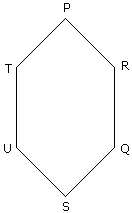# Verbal Reasoning - Seating Arrangement - Discussion

Six friends P, Q, R, S, T and U are sitting around the hexagonal table each at one corner and are facing the centre of the hexagonal. P is second to the left of U. Q is neighbour of R and S. T is second to the left of S.

3.

Which of the following are the neighbours of P ?

 [A]. U and P [B]. T and R [C]. U and R [D]. Data inadequate

Explanation:T and R are the neighbours of P.

 Deep said: (Sep 14, 2014) Option D will be the correct choice as position of S/R/T is not certain. If I am wrong please correct.

 Mohan said: (Jun 9, 2016) No deep the answer is correct. Just read the question carefully watching the diagram. Then you will get it.

 Mallikarjun Bhanje said: (Feb 21, 2020) U and R will be the correct answer.

 Adithyan said: (Oct 9, 2021) Yes, U and R will be the correct answer.